# Airy equation

(diff) ← Older revision | Latest revision (diff) | Newer revision → (diff)

The second-order linear ordinary differential equation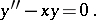It occurred first in G.B. Airy's research in optics . Its general solution can be expressed in terms of Bessel functions of order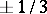: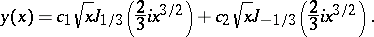Since the Airy equation plays an important role in various problems of physics, mechanics and asymptotic analysis, its solutions are regarded as forming a distinct class of special functions (see Airy functions).

The solutions of the Airy equation in the complex plane,have the following fundamental properties:

1) Every solution is an entire function ofand can be expanded in a power series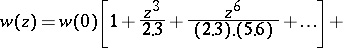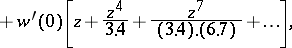which converges for all.

2) If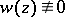is a solution of the Airy equation, then so areand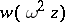, where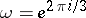, and any two of these solutions are linearly independent. The following identity holds: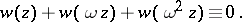How to Cite This Entry:
Airy equation. Encyclopedia of Mathematics. URL: http://encyclopediaofmath.org/index.php?title=Airy_equation&oldid=15069
This article was adapted from an original article by M.V. Fedoryuk (originator), which appeared in Encyclopedia of Mathematics - ISBN 1402006098. See original article